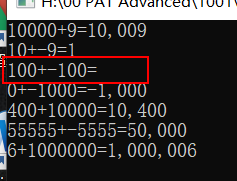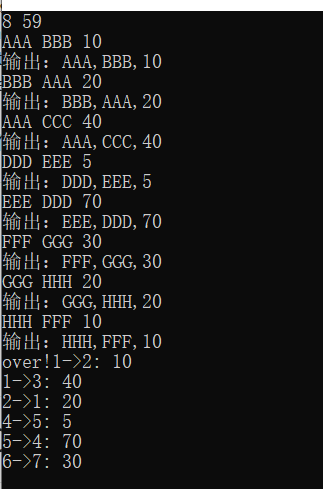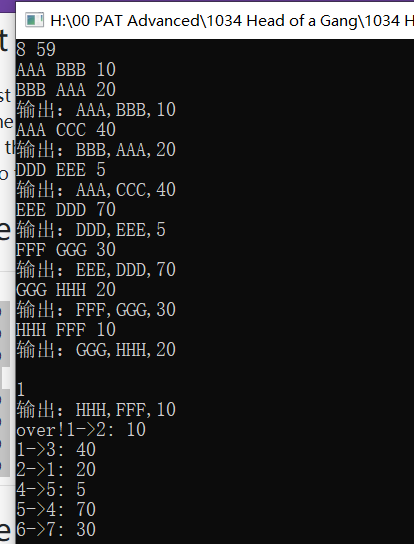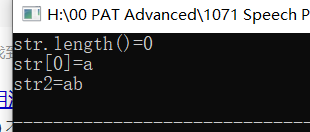# PAT小知识总结

## 注意

• 谨记一句话“所有变量使用前都要初始化赋值。

• 变量尽量全放在开头定义

• 写代码时，设置最大常量比题目要求的数字范围大一点，不然可能提交出现运行时错误（数组边界问题）：

题目要求N (≤1000)

代码设置

const int maxN=1010;

• 得到数组的长度：sizeof(数组名)/sizeof(数组的任一元素)

Java中有方法length()可以直接得到数组的长度；

• 二维数组初始化的赋值

int G[maxN][maxN]= {2};

• 二维数组使用fill：

注意不能写成 fill(G,G+maxN*maxN,INF)

fill(G,G+maxN*maxN,INF);

• fill与memset的区别：

fill 按照单元赋值，将一个区间的元素都赋同一个值

memset 按照字节填充某字符，一般只用于填充char型数据。

参考：

https://blog.csdn.net/liuchuo/article/details/52296646

memset()

https://www.runoob.com/cprogramming/c-function-memset.html

• queue（同理map、vector）中的元素只是复制的副本，某个元素入队后更改该元素的值，不会影响队列内该元素原本的值。

• C++用regex可能会超时，不用

• C++中没有分割字符串函数split()

• 计算c的位数，c不能为0

1+(int)log10(abs(c))

• 1+(int)log10(abs(c))不能计算c=0的情况，需要另外处理解决：

	if(c==0)
i=0;
else if(c<0) //c为负数，加一个符号位
i=(1+(int)log10(abs(c)))+1-1;
else
i=(1+(int)log10(abs(c)))-1;


• 选择更大的数并返回：max(x,y)

• Java里有NULLnull，C++里只有NULL！！

## 输入数据

• scanf匹配数据时，能够自动跳过空白符（空格、回车、制表符）

• string变量不能直接用scanf输入数据，需要先resize预分配空间；或直接用char[]。

*不建议用scanf输入string，用getline(cin,string) 获取

//错误（不会报错，但运行程序会有问题）
string n1,n2;
int t;
scanf("%s %s %d\n",&n1,&n2,&t);

//正确一
string n1,n2;
n1.resize(3); //需要预分配空间
n2.resize(3);
int t;
scanf("%s %s %d\n",&n1,&n2,&t);

//正确二
char n1,n2;
int t;
scanf("%s %s %d\n",&n1,&n2,&t);


• scanf输入数据注意：

• 这样的运行结果：（正常）
scanf("%d%d\n",&N,&K);
for(int i=0; i<N; i++) {
string n1,n2;
int t;
cin>>n1>>n2;
scanf("%d",&t);//最后没加\n
....
}• 这样的运行结果：（错误）需要再打个字符才能继续
scanf("%d%d\n",&N,&K);
for(int i=0; i<N; i++) {
string n1,n2;
int t;
cin>>n1>>n2;
scanf("%d\n",&t);//最后加了\n
....
}## map

• map::end()指向map最后元素之后的地址。

map::find()未找到元素则返回map::end()

## vector

• 对vector进行数组赋值前，必须声明resize！

或在定义vector 就声明长度vector<int> Adj[maxN](maxN);

//resize()
for(int i=0; i<maxN; i++) {
}

scanf("%d",&Adj[j][i]);


*定义vector时声明长度和resize()的作用相同。

• 比较两个vector是否相等、大小，可以用“==”、“>=”、“<=”等

## sort

• 用sort()对vector<vector<int>>排序
• sort()参数写ans.begin()和ans.end()。
• sort可以直接使用greater<vector<int>>()对vector进行排序。
• 自己写比较器时，注意比较器的写法
#include<iostream>
#include<vector>
#include<algorithm>

using namespace std;

vector<int> v1= {10,5,2,7};
vector<int> v2= {10,3,3,6,2};
vector<int> v3= {10,3,3,6,2};
vector<int> v4= {10,4,10};
vector<vector<int>> ans;

bool cmp(vector<int> v1,vector<int> v2) {
return v1>v2;
}

//比较器的错误写法
//bool cmp(vector<int> v1,vector<int> v2) {
//	if(v1>v2) return v1>v2;
//	else return v1<v2;
//}

int main() {
ans.push_back(v1);
ans.push_back(v2);
ans.push_back(v3);
ans.push_back(v4);
//	sort(ans,ans+2*2,cmp);//错误：取到了一个vector，是对象
sort(ans.begin(),ans.end(),cmp);//取到了一个迭代器//方一
//	sort(ans.begin(),ans.end(),greater<vector<int>>());//方二
for(int i=0; i<ans.size(); i++) {
vector<int> temp=ans[i];
for(auto x:temp)
cout<<x<<" ";
cout<<endl;

}

return 0;
}


## string

• string不能单个字符赋值！

string str,str2;

str='a';
str='b';

cout<<"str.length()="<<str.length()<<endl;
cout<<"str="<<str<<endl;

str2="a";
str2+='b';
cout<<"str2="<<str2<<endl;• string::at()返回值为char&类型

• string::insert(下标i，字符串) //字符串插在字符串的下标i后

• 数据类型转换

• string::to_string(c)//int转string

字符串是从第一个字符开始存的： （c=-999,991）cString.at(0)是-

• string::insertStr(1,cString.at(i)) //char转为string

## 结构体struct

• 有关结构体struct：

• 定义结构体时，不能定义自身类型的变量（会导致循环定义），但可以定义自身类型的指针变量。
• 另外声明结构体变量的两种方法
#include<iostream>

using namespace std;

struct node {
int data;
node* child;//不能定义node,但可以定义node*
};

int main() {
/*方一*/
node* childN=new node();
childN->data=2;
cout<<childN->data<<endl;

/*方二*/
//	struct node childN;
//	childN.data=2;
//	cout<<childN.data<<endl;

return 0;
}

posted @ 2020-02-10 14:53  musecho  阅读(...)  评论(...编辑  收藏